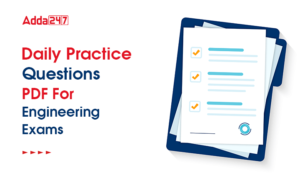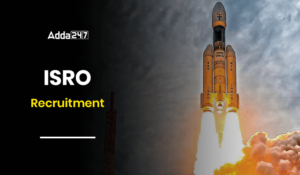Engineering Jobs   »   Quiz: Mechanical Engineering 24 April 2021

# Quiz: Mechanical Engineering 24 April 2021

Quiz: Mechanical Engineering
Exam: GATE
Topic: Miscellaneous

Each question carries 2 mark
Negative marking: 1/3 mark
Time: 20 Minutes

Q1. Under optimal conditions of the process the temperature experienced by a copper work piece in fusion welding, brazing and soldering are such that
(a) T_welding>T_soldering>T_brazing
(b) T_Soldering>T_weolding>T_brazing
(c) T_brazing>T_welding>T_soldering
(d) T_welding>T_brazing>T_soldering

Q2. A cylindrical job with diameter of 200 mm and height of 100 mm is to be cast using modulus method of riser design. Assume that the bottom surface of cylinder riser does not contribute as cooling surface. If the diameter of the riser is equal to its height, then the height of the rise [ in mm] is
(a) 150
(b) 200
(c) 100
(d) 125

Q3. For turning NiCr alloy steel at cutting speeds of 64 m/min and 100 m/min. the respective tool lives are 15 min and 12 min. the tool life for a cutting speed of 144 m/min is
(a) 8 min
(b) 9 min
(c) 10 min
(d) 11.5 min

Q4. A reversible heat engine receives 2 kJ of heat from a reservoir at 1000 K and a certain amount of heat from a reservoir at 800 K. it rejects 1 kJ of heat to a reservoir at 400 K. the net work output (in kJ) of the cycle is
(a) 0.8
(b) 1.0
(c) 1.4
(d) 2.0

Q5. Little’s law is a relationship between
(a) stock level and lead time in an inventory system
(b) waiting time and length of the queue in a queuing system
(c) number of machines and job due dates in a scheduling problem
(d) uncertainty in the activity time and project completion time

Q6. In a CNC feed drive, a steeper motor with step angle of 1.8 degree drives a lead screw with pitch of 2 mm. the basic length unit (BLU) for this drive is
(a) 10 microns
(b) 20 microns
(c) 40 microns
(d) 100 microns

Q7. In spot pulsed laser welding of aluminum plates (density = 2700 kg/m³, specific heat = 896 J/kg, melting temperature = 933 K, latent heat of melting = 398 kJ/kg) at a temp of 30°C, pulse with energy of 0.5 J is focused onto an area of 0.05 mm². if the entire energy is coupled into the material, what will be the depth of weld assuming the CS area of the weld is circular and is uniform throughout its depth and only heat conduction in the direction of penetration
(a) 5.34 mm
(b) 2.15 mm
(c) 4.23 mm
(d) 3.85 mm

Q8. Horse power and kW required per tonne of refrigeration is expressed as
(a) 4.75/COP and 3.5/COP
(b) 3.75/COP and 475/COP
(c) 4.75×COP and 3.5×COP
(d) 4.5/COP and 3.75/COP

Q9. The number of customers arriving at a railway reservation counter is Poisson distributed with an arrival rate of eight customers per hour. The reservation clerk at this counter takes six minutes per customer on an average with an exponentially distributed service time. The average number of the customers in the queue will be
(a) 3
(b) 3.2
(c) 4
(d) 4.2

Q10. A machine element is subjected to the following bi-axial state of stress σ_x=80 MPa; σ_y = 20 MPa; τ_xy = 40 MPa. If the shear strength of the materials is 100 MPa, the factor of safety as per Tresca’s maximum shear stress theory is
(a) 1.0
(b) 2.0
(c) 2.5
(d) 3.3

Solutions
S1. Ans.(d)
Sol. Under the optimal conditions of the process the temperatures experienced by a copper work place is given as
T_welding>T_brazing>T_soldering

S2. Ans.(a)
Sol. Modules method of riser design
M_R=1.2 M_C
(V/A_S )_R=1.2 (V/A_S )_C
If diameter of riser = height of riser
∴ D = 6 M_C
D = 6 [(x/4×(200)^2×100)/(2×π/4×200^2+π×200×100)]
D = (6×200×100)/(400×400)
= 150 mm

S3. Ans.(c)
Sol. V_1=64 ,V_2=100,T_1=15 ,T_1=12
V_1 T_1^h=V_2 T_1^n
V_1 \/V_2=[T_2 \/T_1 ]^n
Taking log on both side
η=(ln V_2 \/V_1)/(ln T_2 \/T_1 )=(ln (100\/64))/(ln (15\/12) )=0.4463/0.2231=2
V_1 T_1^n=V_3 T_3^n
T_3=T_1 (V_1/V_3 )^(1\/n)=15 (64/144)^0.5=10min
Note – strictly speaking the value of n < 1

S4. Ans.(c)
Sol. for reversible heat engine,

(∆s)_(Reversible cycle)=0
θ_1/T_1 +θ_2/T_2 -θ_3/T_3 =0
Or,θ/1000+θ_2/800-1/400=0
θ_2=0.4 kJ
From heat balance
θ_1+θ_2=W+θ_3
2+0.4=W+1
W=1.4 kJ

S5. Ans.(b)
Sol. little’s law is a relationship between waiting time and length of queue in a queuing system.

S6. Ans.(a)
Sol. Pitch = 2mm, step angle = 1.8°/pulse
Distance travelled in one rev (360°) = 2mm
No. of pulses in 360° = (360°)/1.8=200 pulses
Distance travelled in one pulse = 2/200 mm
=0.01 mm
≈ 10 μm

S7. Ans.(d)
Sol. Heat supplied by laser pulse = 0.5 J, consider, depth of weld be x mm.
Heat consumed = heat taken to increase the flame + heat taken to melt the material
0.5=0.05x×2700/109 [896(933-303)+398×10^3 ] J
x=3.84 mm

S8. Ans (a)
Sol. We know that
COP=(Refrigeration effect in ton)/(external power given )
External power given in kW =3.5/COP (If refrigeration effect=1 ton) and (1 ton=3.5 kW)
External power given in HP =4.75/COP (If refrigeration effect=1 ton) and (1 ton=4.78 HP)

S9. Ans.(b)
Sol. Arrival rate (λ) = 8 per hour
Service rate (μ) = 60/6 = 10 per hour
Average no. of customer in the queue
L_q=λ^2/(μ(μ-λ) )=(8)^2/10(10-8) =3.2

S10. Ans.(b)
Sol.
σ_1,2=1/2 [(σ_x+σ_y )±√((σ_x-σ_y )^2+4τ_xy^2 ) ]
=1/2 [(80+20)±√((60)^2+4×40^2 ) ]
σ_1=100 MPa
σ_2=0 MPa
τ_max=σ_1/2=50 MPa
f(factor of safety)=τ_ys/τ_max =100/50=2

Sharing is caring!

•GATE Result 2023 Out, Download Result, C...
•GATE 2023 Admit Card Out, Download Link ...
•UKPSC Draftsman Syllabus 2023 and Exam P...
•UP Polytechnic Exam Date 2023 Out, Downl...
•JSSC JE Syllabus 2023, Check Latest Exam...
•Daily Practice Questions PDF For Enginee...
•ISRO Recruitment 2023, Latest Notificati...
•UPSC ESE Previous Year Papers, Download ...